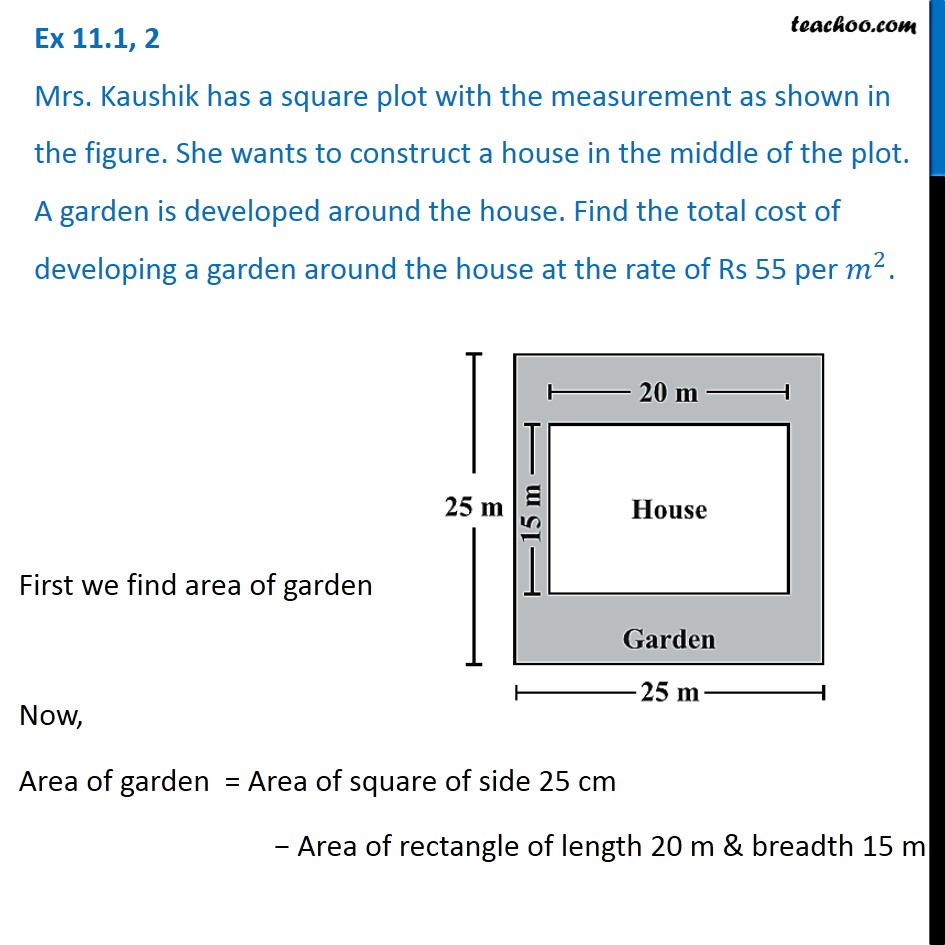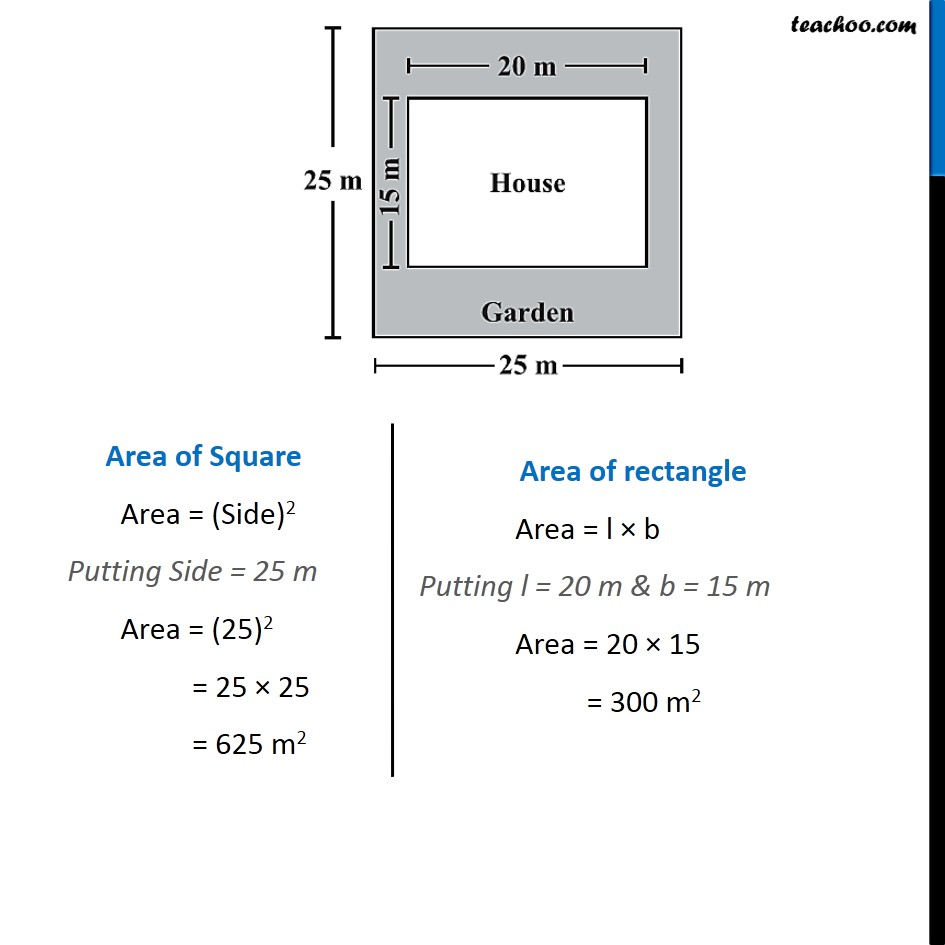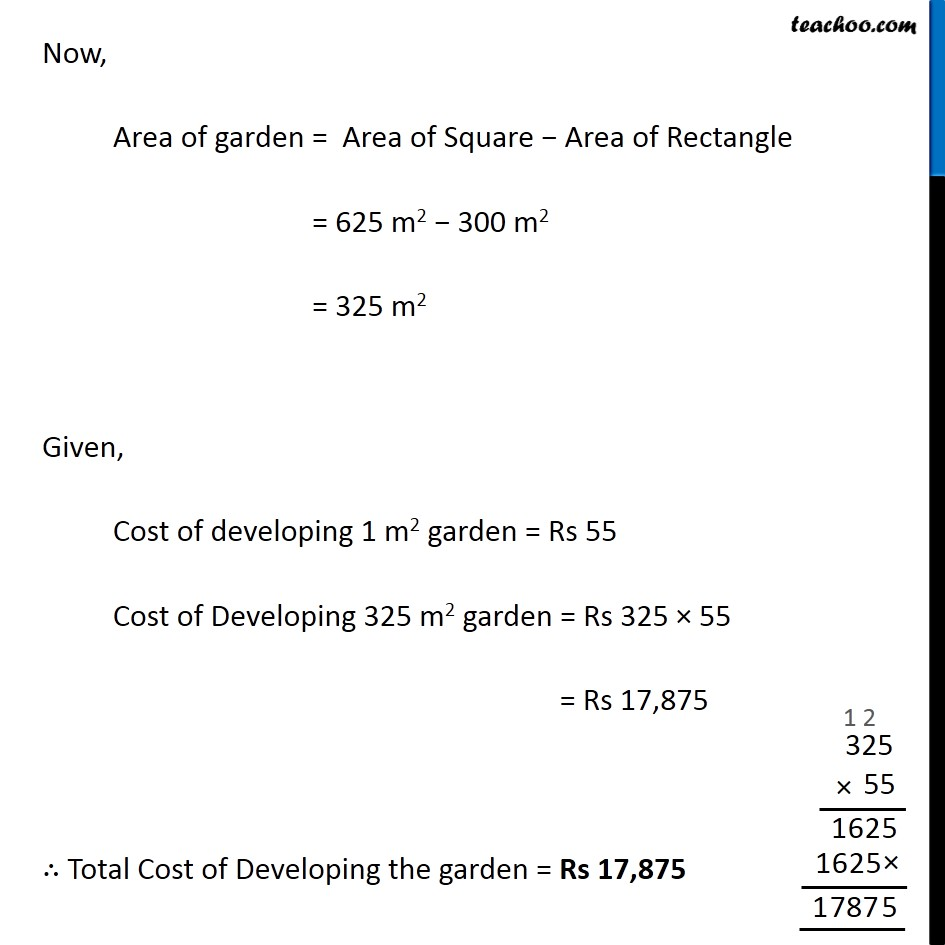Area and Perimeter

Chapter 9 Class 8 Mensuration
Concept wiseLearn in your speed, with individual attention - Teachoo Maths 1-on-1 Class

### Transcript

Question 2 Mrs. Kaushik has a square plot with the measurement as shown in the figure. She wants to construct a house in the middle of the plot. A garden is developed around the house. Find the total cost of developing a garden around the house at the rate of Rs 55 per 𝑚^2. First we find area of garden Now, Area of garden = Area of square of side 25 cm − Area of rectangle of length 20 m & breadth 15 m Area of Square Area = (Side)2 Putting Side = 25 m Area = (25)2 = 25 × 25 = 625 m2 Area of rectangle Area = l × b Putting l = 20 m & b = 15 m Area = 20 × 15 = 300 m2 Now, Area of garden = Area of Square − Area of Rectangle = 625 m2 − 300 m2 = 325 m2 Given, Cost of developing 1 m2 garden = Rs 55 Cost of Developing 325 m2 garden = Rs 325 × 55 = Rs 17,875 ∴ Total Cost of Developing the garden = Rs 17,875